OEImageBase¶

class OEImageBase

The OEImageBase is an abstract class that provides methods for drawing basic geometric shapes such as lines, circles, rectangles etc.

The following classes derive from this class:

Constructors¶

OEImageBase(double width=200.0, double height=200.0)

Default constructor that creates an image with the specified width and height.

width, height

The dimensions of the image, both have to be positive (non-zero) numbers.

Clear¶

void Clear(const OESystem::OEColor &color=OESystem::OEWhite)

Clears the image with the given color.

DrawArc¶

void DrawArc(const OE2DPoint &center, double bgnAngle, double endAngle,

Draws the outline of an arc. See example in Figure: Example of arc.

center

The center of the arc.

bgnAngle, endAngle

The two endpoints of the arc (in degrees). Both angles are in degrees and their values have to be in a range of $$[0.0^{\circ}, 360.0^{\circ}]$$. Angles are interpreted such that $$0.0^{\circ}$$ and $$360.0^{\circ}$$ degrees are at the 12 o’clock position, $$90.0^{\circ}$$ degrees corresponds to 3 o’clock, etc.

pen

The graphical properties of the curve (such as color, line width, etc). See examples in Figure: Example of drawing arcs with various pens.

Note

OEImageBase.DrawArc supports all the OEPen properties (i.e. color, line width, line stipple) except the fill property. See also OEImageBase.DrawPie method.

Arcs are always drawn in a clockwise direction. This means that bgn = $$0.0^{\circ}$$, end = $$90.0^{\circ}$$ angle pair is not the same as bgn = $$90.0^{\circ}$$, end = $$0.0^{\circ}$$ angle pair. See examples in Table: Example of interpreting the begin and end angles of the arc.

Example of interpreting the begin and end angles of the arc

bgn = $$0.0^{\circ}$$ end = $$90.0^{\circ}$$

bgn = $$90.0^{\circ}$$ end = $$0.0^{\circ}$$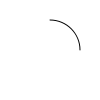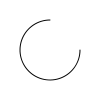DrawCircle¶

void DrawCircle(const OE2DPoint &center, double radius, const OEPen &pen)

Draws a circle. See example in Figure: Example of circle.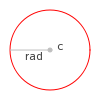Example of circle

center

The center of the circle.

pen

The graphical properties of the circle (such as color, line width, etc). See examples in Figure: Example of drawing circles with various pens.

DrawCubicBezier¶

void DrawCubicBezier(const OE2DPoint &bgn, const OE2DPoint &c1,
const OE2DPoint &c2, const OE2DPoint &end, const OEPen &pen)

Draws a cubic Bézier curve. See example in Figure: Example of cubic Bezier curve.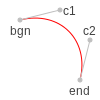Example of cubic Bezier curve

bgn, end

The end points of the Bezier curve.

c1, c2

The control points of the curve.

pen

The graphical properties of the Bézier curve (such as color, line width, etc). See examples in Figure: Example of drawing cubic Bezier curves with various pens.

image = oedepict.OEImage(100, 100)

b = oedepict.OE2DPoint(20, 70)
e = oedepict.OE2DPoint(60, 70)
c1 = b + oedepict.OE2DPoint(50, -60)
c2 = e + oedepict.OE2DPoint(50, -60)

pen = oedepict.OEPen(oechem.OELightGreen, oechem.OEBlack, oedepict.OEFill_On, 2.0)
image.DrawCubicBezier(b, c1, c2, e, pen)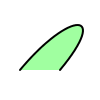Example of drawing a cubic Bezier curve

DrawLine¶

void DrawLine(const OE2DPoint &bgn, const OE2DPoint &end, const OEPen &pen)

Draws a line. See example in Figure: Example of line.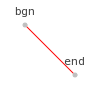Example of line

bgn, end

The two endpoints of the line.

pen

The graphical properties of the line (such as color, line width, etc). See examples in Figure: Example of drawing lines with various pens.

DrawPath¶

void DrawPath(const OE2DPath& path, const OEPen& pen);

Draws as path. See example in Figure: Example of path.

path

The OE2DPath object that defines a path as a sequence of lines and curves.

pen

The graphical properties of the pie (such as color, line width, etc). See examples in Figure: Example of drawing paths with various pens.

Example (Figure: Example of drawing a path)

image = oedepict.OEImage(100, 100)

path = oedepict.OE2DPath(oedepict.OE2DPoint(20, 80))
oedepict.OE2DPoint(20, 40))

pen = oedepict.OEPen(oechem.OELightGreen, oechem.OEBlack, oedepict.OEFill_On, 2.0)
image.DrawPath(path, pen)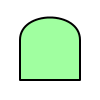Example of drawing a path

DrawPie¶

void DrawPie(const OE2DPoint &center, double bgnAngle, double endAngle,

Draws a pie. See example in Figure: Example of drawing a pie.

center

The center of the pie.

bgnAngle, endAngle

The two endpoints of the pie (in degrees). Both angles are in degrees and their values have to be in a range of $$[0.0^{\circ}, 360.0^{\circ}]$$. Angles are interpreted such that $$0.0^{\circ}$$ and $$360.0^{\circ}$$ degrees are at the 12 o’clock position, $$90.0^{\circ}$$ degrees corresponds to 3 o’clock, etc.

pen

The graphical properties of the pie (such as color, line width, etc). See examples in Figure: Example of drawing pies with various pens.

Pies are always drawn in a clockwise direction. This means that bgn = $$0.0^{\circ}$$, end = $$90.0^{\circ}$$ angle pair is not the same as bgn = $$90.0^{\circ}$$, end = $$0.0^{\circ}$$ angle pair. See examples in Table: Example of interpreting the begin and end angles of the pie.

Example of interpreting the begin and end angles of the pie

bgn = $$0.0^{\circ}$$ end = $$90.0^{\circ}$$

bgn = $$90.0^{\circ}$$ end = $$0.0^{\circ}$$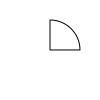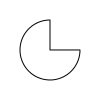DrawPoint¶

void DrawPoint(const OE2DPoint &p, const OESystem::OEColor &color) = 0

Draws a point. See example in Figure: Example of point.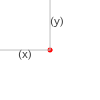Example of point

p

The position of the point, where p = OE2DPoint(x, y).

color

The color of the point.

DrawPolygon¶

void DrawPolygon(const std::vector<OE2DPoint> &points, const OEPen &pen)

Draws a closed polygon. See example in Figure: Example of polygon.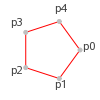Example of polygon

points

The series of points that defines the polygon. The polygon will be closed i.e. the first connected with the last point.

pen

The graphical properties of the polygon (such as color, line width, etc). See examples in Figure: Example of polygons lines with various pens.

Example (Figure: Example of drawing a polygon)

image = oedepict.OEImage(100, 100)

polygon = []
polygon.append(oedepict.OE2DPoint(20, 20))
polygon.append(oedepict.OE2DPoint(40, 40))
polygon.append(oedepict.OE2DPoint(60, 20))
polygon.append(oedepict.OE2DPoint(80, 40))
polygon.append(oedepict.OE2DPoint(80, 80))
polygon.append(oedepict.OE2DPoint(20, 80))

pen = oedepict.OEPen(oechem.OELightGreen, oechem.OEBlack, oedepict.OEFill_On, 2.0)
image.DrawPolygon(polygon, pen)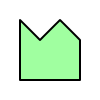Example of drawing a polygon

void DrawQuadraticBezier(const OE2DPoint &bgn, const OE2DPoint &c,
const OE2DPoint &end, const OEPen &pen)

Draws a quadratic Bézier curve. See example in Figure: Example of quadratic Bezier curve.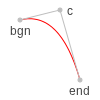bgn, end

The end points of the Bézier curve.

c

The control point of the curve.

pen

The graphical properties of the Bézier curve (such as color, line width, etc). See examples in Figure: Example of drawing quadratic Bezier curves with various pens.

Example (Figure: Example of drawing a polygon)

image = oedepict.OEImage(100, 100)

b = oedepict.OE2DPoint(20, 70)
e = oedepict.OE2DPoint(80, 70)
c = b + oedepict.OE2DPoint(30, -80)

pen = oedepict.OEPen(oechem.OELightGreen, oechem.OEBlack, oedepict.OEFill_On, 2.0)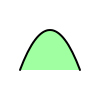Example of drawing a quadratic Bezier

DrawRectangle¶

void DrawRectangle(const OE2DPoint &tl, const OE2DPoint &br, const OEPen &pen)

Draws a rectangle. See example in Figure: Example of rectangle.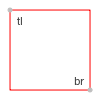Example of rectangle

tl

The top left corner of the rectangle.

br

The bottom right corner of the rectangle.

pen

The graphical properties of the rectangle (such as color, line width, etc). See examples in Figure: Example of polygons rectangles with various pens.

DrawText¶

void DrawText(const OE2DPoint &center, const std::string &text, const OEFont &font,
double maxwidth=0.0)

Draws a text. See example in Figure: Example of text.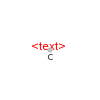Example of text

center

The position of the text.

text

The characters to be displayed.

font

The graphical properties of the displayed text (such as color, font size, etc). See examples in Figure: Example of drawing texts with various fonts.

maxwidth

If maxwidth is specified and the width of the text would exceed the given limit, than the size of the font used to display the text is automatically reduced.

Note

The displayed position of the text not only depends on ‘c’ position but also the alignment style of the given OEFont.

DrawTriangle¶

void DrawTriangle(const OE2DPoint &a, const OE2DPoint &b, const OE2DPoint &c,
const OEPen &pen)

Draws a triangle. See example in Figure: Example of triangle.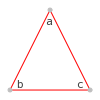Example of triangle

a, b, c

The three corners of the triangle.

pen

The graphical properties of the triangle (such as color, line width, etc). See examples in Figure: Example of polygons triangles with various pens.

GetGlobalOffset¶

const OE2DPoint& GetGlobalOffset() const

Returns the offset from the origin (x=0.0, y=0.0) to the top-left corner of the given image.

GetHeight¶

double GetHeight() const

Returns the height of the OEImageBase object.

GetMinFontSize¶

unsigned int GetMinFontSize() const

Returns the minimum size of the font that can be displayed.

GetWidth¶

double GetWidth() const

Returns the width of the OEImageBase object.

SetMinFontSize¶

void SetMinFontSize(unsigned int fontsize)

Sets the minimum size of the font that can be displayed.

GetSVGClass¶

OESVGClass *GetSVGClass(const std::string &name)
const OESVGClass *GetSVGClass(const std::string &name) const

Returns the OESVGClass object with the given name. Null pointer will be returned if no class exists on the image with the given name.

GetSVGGroup¶

OESVGGroup *GetSVGGroup(const std::string &id)
const OESVGGroup *GetSVGGroup(const std::string &id) const

Returns the OESVGGroup object with the given name. Null pointer will be returned if no group exists on the image with the given name.

GetSVGGroups¶

OESystem::OEIterBase<OESVGGroup>* GetSVGGroups()

Returns an iterator over the SVG groups (OESVGGroup) of the image.

Example:

for g in image.GetSVGGroups():
print(g.GetId())

NewSVGClass¶

OESVGClass *NewSVGClass(const std::string &name)

Creates a new OESVGClass on the given image. A null pointer will be returned and warning message will be thrown if the SVG class can not be created.

name

The name of the OESVGClass object. For information about valid group names see the documentation of the OEIsValidSVGClassName function.

NewSVGGroup¶

OESVGGroup *NewSVGGroup(const std::string &id)

Creates a new OESVGGroup on the given image. A null pointer will be returned and warning message will be thrown if the SVG group can not be created.

name

The name of the OESVGGroup object. For information about valid group names see the documentation of the OEIsValidSVGGroupId function.

PopGroup¶

bool PopGroup(const OESVGGroup *)

Pops the OESVGGroup object.

Note

An OESVGGroup object can be used (pushed / popped) only once. OESVGGroup objects can be nested. They should be used in LIFO (for last in, first out) i.e. the group that has been pushed last should be popped first.